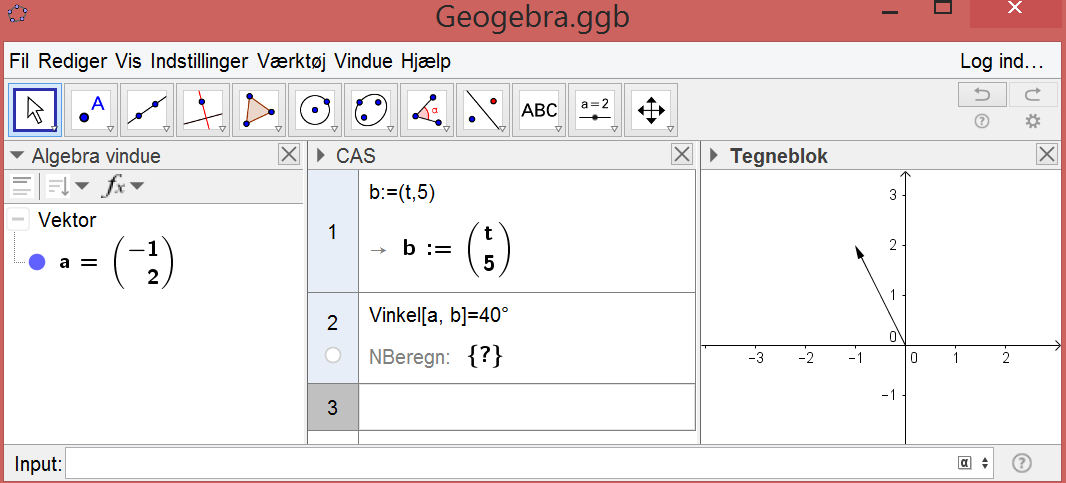# Equation "angle between 2 vectors" in CAS

Fritzbøger shared this problem 5 years ago
Solved

It Works in 3D but not in 2D?

Vinkel is angle in danish1

As a workround you can also try solving the equation

Angle[a,(x(b),y(b))]=40deg

(because (x(b),y(b)) converts vector to a point)1

Thank you.

angle[a,B]=40 deg

This Works if B:=(t,5) in CAS. Same solution as yours.1

It is solved now. Thank you.